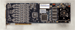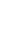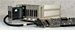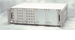# Data Acquisition Glossary#### A  -  B  -  C  -  D  -  E  -  F  -  G  -  H  -  I  -  J  -  K  -  L  -  M  -  N  -  O  -  P  -  Q  -  R  -  S  -  T  -  U  -  V  -  W  -  X  -  Y  -  Z

accelerometer
a sensor that responds to acceleration, often using a piezo crystal that generates a voltage when distorted
accuracy
a stated maximum deviation from the NIST standard
acquisition speed
analog-to-digital conversion speed
active filter
a filter that incorporates active components, usually as amplifiers, so that it more closely approximates an ideal filter
actuator
a device that converts an electrical signal into a physical quantity
A/D conversion
analog-to-digital conversion
ADC
analog-to-digital converter
alias frequency
a false signal component in a stream of data corrupted by sampling frequencies above Nyquist
aliasing
the presence of alias frequencies in acquired data
analog-to-digital conversion (A/D conv.)
sampling voltage at a given moment and digitizing the value
analog-to-digital conversion time
the time taken to convert one sample, the inverse of the sampling rate (acquisition speed), with 4 microseconds (time), for example, corresponding to 250k samples per second (rate).
analog-to-digital converter ( ADC)
hardware component that samples voltage and digitizes the measured value
antialiasing filter
analog filter employed to remove frequencies above Nyquist, because these high-frequency signal components corrupt acquired data and, although digital filters perform better than analog filters at many tasks, digital processing cannot remove frequencies above Nyquist
bipolar
either terminal may have a higher voltage than the other
bridge
a resistor bridging the junctions of two pairs of voltage-dividing series resistors, and that carries no current when the two pairs divide the voltage equally
buffer
temporary storage for data values in a process - with the additional sense, in hardware, of isolation or protection
burst mode
processing at high speed, usually to or from a buffer
calibration
adjusting equipment to improve its accuracy
calibration period
the interval between calibrations, usually one year for data acquisition equipment
channel
a signal or data path
CJC
cold-junction compensation
CMR
common mode rejection
CMRR
common mode rejection ratio
cold junction
the constant-temperature reference junction of a thermocouple, classically in water kept at freezing point by thawing ice
cold-junction compensation (CJC)
circuitry that allows for an ambient-temperature reference junction
common mode input voltage
how far signal ground may differ from system ground for differential inputs
common mode rejection (CMR)
the rejection of a common mode signal, often noise, applied equally to both inputs and that ideally results in no differential signal
common mode rejection ratio (CMRR)
a measure of common mode rejection, and therefore, ideally, of the ability to reject noise
common-mode signal
a signal applied simultaneously and equally at both differential inputs
continuous acquisition
acquiring data without having to pause to prevent buffer overflow
conversion rate
the inverse of conversion time, with a conversion rate of 250k samples per second corresponding to a conversion time of 4 microseconds, for example
conversion time
analog-to-digital conversion time or digital-to-analog conversion time
counter
a circuit that counts pulses; also, in software, the address of a count
counter/timer Input
a digital input that channels a signal to high-speed hardware that counts pulses or measures frequencies
cut-off frequency
the highest frequency that can pass through a filter without attenuation
D/A conversion
digital-to-analog conversion
DAC
digital-to-analog converter
data acquisition
converting an analog signal into a time series of digital values, with each value recording the signal voltage at that instant
more data acquisition terms...
data reduction
summarizing a stream of data, often in real time, to extract useful information - such as blocks of data surrounding peaks or triggers
datalogger
a device that records acquired data for later collection
differential amplifier
a cicuit that amplifies the difference between two inputs
differential inputs
a signal input and its individual ground
digital signal processing (DSP)
processing analog signals in the digital domain: performing mathematical operations on digitized samples of a signal to achieve results -- filtering, phase-shifting -- previously obtainable only by passing an analog signal through special circuits
digital-to-analog conversion (D/A conv.)
recreating an analog signal from a series of digital values
digital-to-analog converter (DAC)
a device that creates an analog signal level from a digital value
DMA
direct memory access: a data transfer between memory and a peripheral that bypasses the CPU
DSP
digital signal processing
dynamic range
the ratio of full-scale to the smallest measurable unit: 96dB in a 16-bit instrument (6dB per bit of resolution)
event counter
a circuit that counts pulses
excitation
a voltage or current required to operate actuators and some sensors
external trigger
a pulse on a control line that triggers sampling on other channels
FFT (fast Fourier transform)
a transform of signal data from time to frequency domains: an algorithm that analyzes a finite time series of data points into component frequencies
filtering
attenuating unwanted frequency components of a signal
frequency counter
a circuit that counts pulses within a specified time, the gate time, often one second or less

G - H - I - J - K    (return to top)

gain
amplification factor
hardware trigger
an external trigger; see also 'software trigger'
IA
instrumentation amplifier
instrumentation amplifier (IA)
a high-impedance differential amplifier with high common-mode rejection
isolation amplifier
an instrumentation amplifier with electrically isolated inputs

L - M - N    (return to top)

LSB
Least Significant Bit: the rightmost bit, bit zero, with weight 2 to the zeroth power (equal to one), in a binary representation of a value
LVDT
Linear Variable Differential Transformer: a sensor that requires AC excitation of a primary coil to output AC from a secondary coil with amplitude that varies with position of a movable core
MSB
Most Significant Bit: the leftmost bit, bit N-1 in an N-bit binary representation, with weight 2 to the (N-1)th power
Nyquist frequency
half the sampling rate, the frequency above which data acquisition fails because samples of higher frequency signal components appear in the data stream as if sampled from lower frequency components that do not, in fact, exist: alias frequencies

O - P - Q    (return to top)

offset
an added constant, a zero-correction
optical isolation
optical signal transmission between electrically isolated networks
PID
Proportional Integral Derivative control algorithm that moderates a proportional gain with integral and derivative time factors to hold a measurement or process variable at or near a set point
programmable gain
amplification of a signal by specific gains under software control
quadrature encoder
circuit emitting two pulse streams generated by a rotating shaft that another special circuit can decode to reveal shaft position and direction of rotation
real-time processing
processing a stream of data during acquisition: scaling, transforming, reducing, filtering or performing other operations on data, rather than simply streaming unprocessed data to disk for subsequent analysis
resolution
a measure of the least possible change in relation to full scale: 10000/4096=2.44mV, for example, at 12-bit resolution when 10V represents full scale
sample and hold (S/H)
storing a sampled analog voltage on a capacitor for a short period
sample rate
the rate of sampling in samples, kilosamples, or megasamples per second
sampling
taking the value of signal, usually as a voltage, and then, typically, digitizing it
scaling
applying a factor and an offset to a signal
sensitivity
minimum detectable signal change
sensor
a device that converts a physical quantity into an electrical signal
set point
value of a variable that a controller maintains by responding to errors
settling time
the time for an applied change in input voltage to result in a changed output voltage (stable within specified limits)
signal
information carried in some medium, often a waveform
signal conditioning
preparing an analog signal before digitizing it: amplifying the signal, removing frequencies above half the sampling rate (the Nyquist frequency), linearizing it, and so on, although digital processing now can perform linearization and other traditional signal conditioning tasks more effectively than analog circuitry
signal-to-noise ratio (SNR)
rms of signal to rms of noise, expressed in decibels
simultaneous sample and hold
using a clock to strobe several channels into sample and hold circuits
simultaneous sampling
digitizing the values from simultaneous sample and hold operations
single-ended input
a signal channel that shares a common ground with one or more other signal channels
slew rate
maximum rate of change of output voltage from an op amp
software trigger
real-time processing and analysis of one or more signals to determine some action by the system
strain gauge
deformation-sensitive resistor bonded to material under test, part of a bridge circuit
successive approximation
digitizing an analog voltage in n comparisons (for n-bit resolution) as though determining the weight of an object with a balance and a decreasing series of binary weights
time stamp
a count attached to a data item to record the units of time elapsed since a reference time
transducer
a sensor or actuator
trigger
a hardware control signal, or real-time processing and analysis of one or more signals by software, that triggers some system action

U - V - W - X - Y - Z    (return to top)

unipolar
one of the terminals, the positive terminal, never has a lower voltage than the other